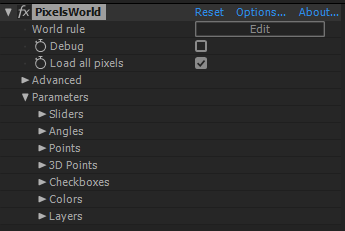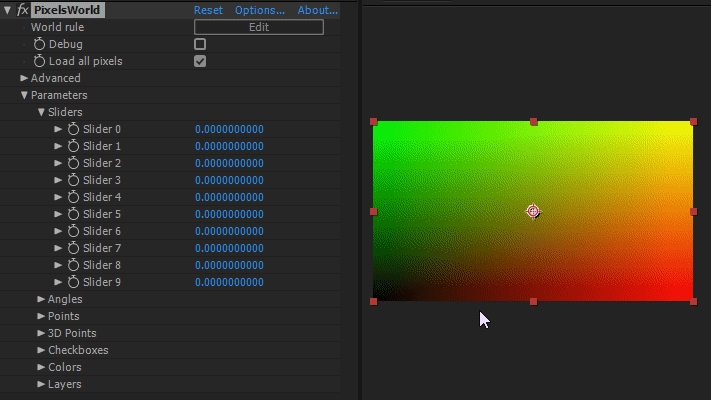# 链接参数• Sliders
• Angles
• Points
• 3D Points
• Checkboxes
• Colors
• Layers

`````` void main(){
outColor = vec4(uv,slider,1.0);
}
``````## 详情

``````uniform float slider;
uniform float angle;
uniform bool checkbox;
uniform vec2 point;
uniform vec3 point3d;
uniform vec4 color;
uniform sampler2D layer;
uniform sampler2D inLayer;
``````

• "inLayer" 代表输入层。
• sampler2D 是一种由 GLSL提供的变量类型，不用理解该变量类型。如果你想了解图层参数，请参考 "getColor"
• "uniform" 代表全局变量。